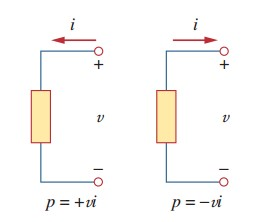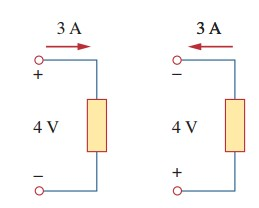omG8HI9LSKFW6lhCj8prTs0Z6lUhdOT9Jhi1Sf4m

# Electric Power Formula

## Electrical Power and Energy

Although current and voltage are fundamental variables for electric circuits, they are not still sufficient to solve all the electrical phenomenon. In fact we still need to know how much power an electronic devices can handle. For example, 120-watt bulb will shine brighter than 60-watt bulb. Another easy example is electricity bills that we pay is based on how much electric energy we used in a certain period of time. Hence, the analysis of power and energy are taking important part besides current and voltage in circuit analysis.

To discover the relationship between energy and power to current and voltage, we have physic statement :
Power is the time rate of expending or absorbing energy, measured in watts (W)
And the mathematic equation is :

where :
p  = power (watts = W)
w = energy (joules = J)
t   = times (seconds = s)

Combining the Equation.(1) with the equation of voltage and equation of current results :

or
Mathematic equation in Equation.(3) is called by instantaneous power. If the power has positive (+) sign, the power is being delivered to element or the element is absorbing power. In opposite negative (-) sign means the power is being supplied by element. These condition can be seen in Figure.(1).Figure 7. Power signs
In order to determine the sign of power we need to consider the current and voltage direction first. For convenient method of this problem we can review the relationship between current, voltage, and power. Please refer to Figure.(1) for better understanding about the relationship between these three parameters.Figure 2. Elemet absorbing power
Figure.(2) shows how to get positive sign. This is known as passive sign convention. This convention shows how the current enters through positive polarity of voltage, makes p = +vi or p > 0 implies that the element is absorbing power. In opposite for p = -vi or vi < 0 in Figure.(3) means the element is delivering or supplying power.Figure 3. Elemet delivering power
Passive sign convention is achieved when the current enters through the positive terminal of an element and p = +vi, if the current enters the negative terminal, p = -vi.
For ilustration above we will conclude that :
+Power absorbed = -Power supplied
because absorbing power of -12W is the same with supplying power of +12W.
We now understand that law of conservation of energy must be obeyed by any electric circuit. The mathematic equation itself may follow :(4)
From equation above it makes sure that the total power absorbed must balance the total power supplied.
Using Equation.(2) but in changing condition in a spesific period of time, the equation transforms into :(5)
Energy is the capacity to do work, measured in joules (J)
The electric power utility measure their energy in watt-hours (Wh) where :
1 Wh = 3.600 J

For better understanding let us review some examples below :

1.An energy sources forces a constant current of 2 A for 10 s to flow to a light bulb. If 2.3 kJ is used as light and heat energy, how much voltage drop for the bulb?
Solution :
2.Calculate the power delivered to an element at t = 3 ms if the current is entering at positive terminal
i =  5 cos 60 π t A
and the voltage is : (a) v = 3i ; and (b) v = 3 di/dt
Solution :

Untuk Bahasa Indonesia baca Definisi Daya Listrik dan Rumus Daya Listrik
Related Posts
SHARE